# Types of Angles

An angle is formed possibly from $0^\circ$ to $360^\circ$ for a rotation. The angles from $0^\circ$ to $360^\circ$ are classified into seven types of angles in geometry. Here is the list of different types of angles with examples.

## List of angles

1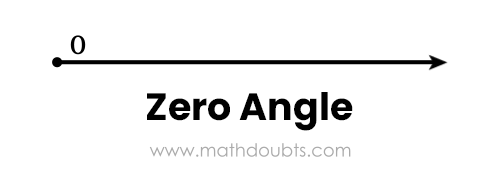### Zero angle

An angle of $0^\circ$.

2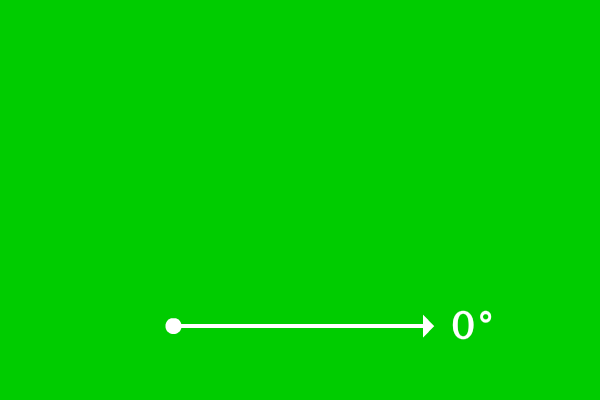### Acute angle

An angle of less than $90^\circ$.

3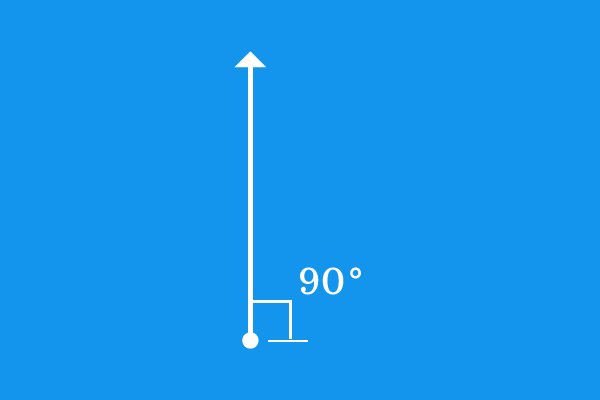### Right angle

An angle of $90^\circ$.

4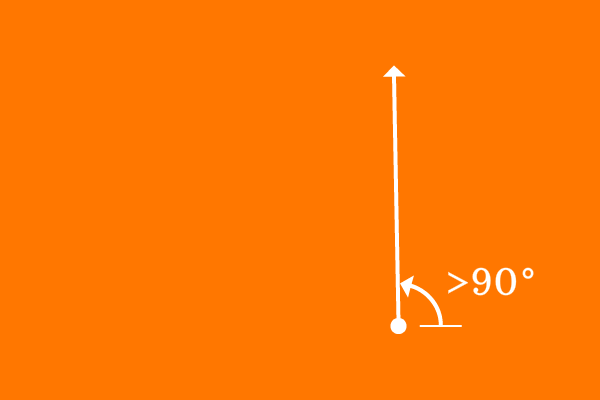### Obtuse angle

An angle of greater than $90^\circ$ and less than $180^\circ$.

5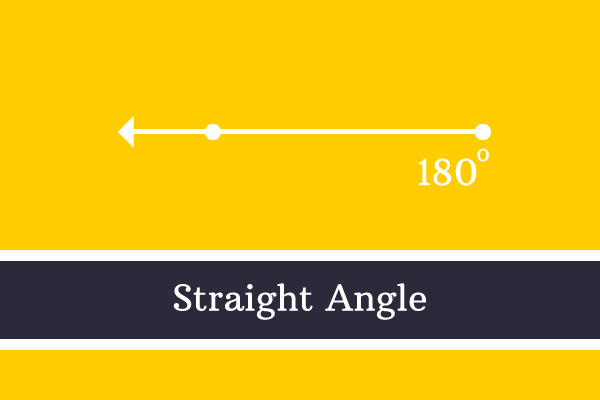### Straight angle

An angle of $180^\circ$.

6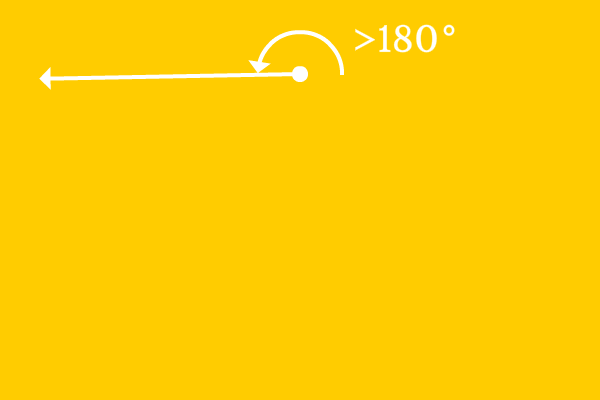### Reflex angle

An angle of greater than $180^\circ$ and less than $360^\circ$.

7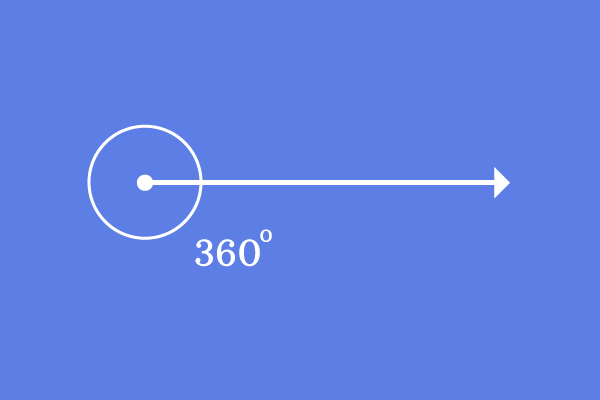### Complete angle

An angle of $360^\circ$.

A best free mathematics education website that helps students, teachers and researchers.

###### Maths Topics

Learn each topic of the mathematics easily with understandable proofs and visual animation graphics.

###### Maths Problems

A math help place with list of solved problems with answers and worksheets on every concept for your practice.

Learn solutions

###### Subscribe us

You can get the latest updates from us by following to our official page of Math Doubts in one of your favourite social media sites.